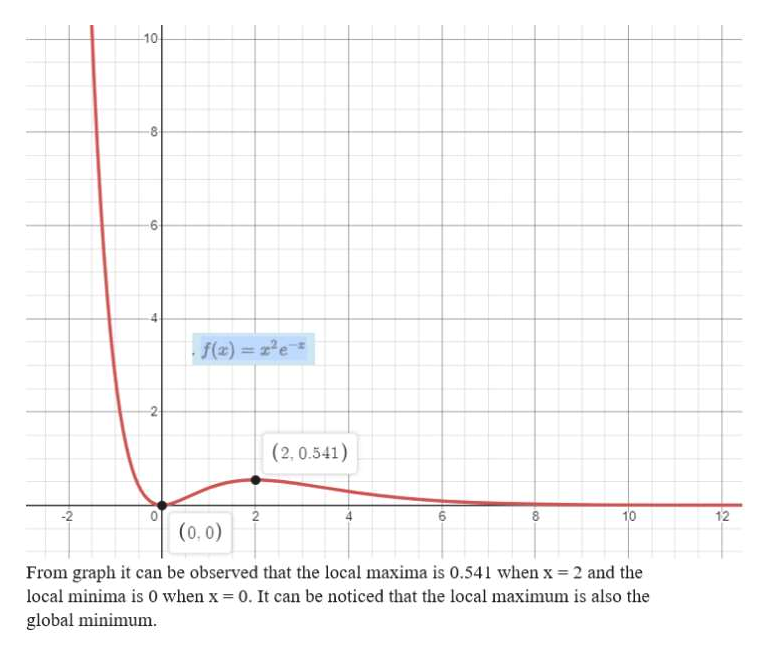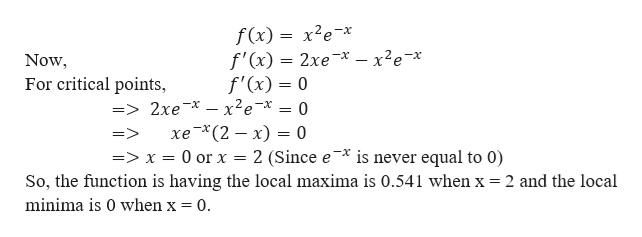Question
68 views
1. Use a graph of  to estimate the maximum and minimum values. Then find the exact values.

2. Estimate the value of  at which  increases most rapidly. Then find the exact value

check_circle

Step 1
1. First, here we will use the graph of the function to estimate the maximum and minimum values.help_outlineImage Transcriptionclose10 8 f(x) ze 2 (2,0.541) -2 10 8 (0,0) From graph it can be observed that the local maxima is 0.541 when x 2 and the local minima is 0 when x 0. It can be noticed that the local maximum is also the global minimum. 12 fullscreen
Step 2

Now we find the exact v...help_outlineImage Transcriptionclosef(x) x2e-* f'(x) 2xe*-x2e* f'(x) = 0 => 2xe xe-* = 0 хе * (2 — х) — 0 = Now For critical points = => 2 (Since ex is never equal to 0) => x = 0 or x So, the function is having the local maxima is 0.541 when x = 2 and the local minima is 0 when x = 0. fullscreen

### Want to see the full answer?

See Solution

#### Want to see this answer and more?

Solutions are written by subject experts who are available 24/7. Questions are typically answered within 1 hour.*

See Solution
*Response times may vary by subject and question.
Tagged in

### Derivative